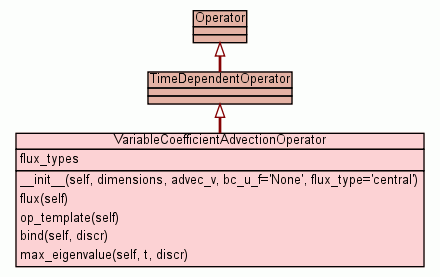[frames] | no frames]

source codeA class for space- and time-dependent DG-advection operators.

`advec_v` is a callable expecting two arguments `(x, t)` representing space and time, and returning an n-dimensional vector representing the velocity at x. `bc_u_f` is a callable expecting `(x, t)` representing space and time, and returning an 1-dimensional vector representing the state on the boundary. Both `advec_v` and `bc_u_f` conform to the `hedge.data.ITimeDependentGivenFunction` interface.

 Instance Methods

 __init__(self, dimensions, advec_v, bc_u_f=`'``None``'`, flux_type=`'``central``'`) x.__init__(...) initializes x; see x.__class__.__doc__ for signature source code

 flux(self) source code

 op_template(self) source code

 bind(self, discr) source code

 max_eigenvalue(self, t, discr) source code

Inherited from `object`: `__delattr__`, `__getattribute__`, `__hash__`, `__new__`, `__reduce__`, `__reduce_ex__`, `__repr__`, `__setattr__`, `__str__`

 Class Variables
flux_types = `['central', 'upwind', 'lf']`
 Properties

Inherited from `object`: `__class__`

 Method Details

### __init__(self, dimensions, advec_v, bc_u_f=`'``None``'`, flux_type=`'``central``'`)(Constructor)

source code

x.__init__(...) initializes x; see x.__class__.__doc__ for signature

Overrides: object.__init__
(inherited documentation)

 Generated by Epydoc 3.0.1 on Sat Aug 29 14:33:32 2009 http://epydoc.sourceforge.net